# 布隆过滤器(BloomFilter)的原理、实现和探究

2019独角兽企业重金招聘Python工程师标准>>>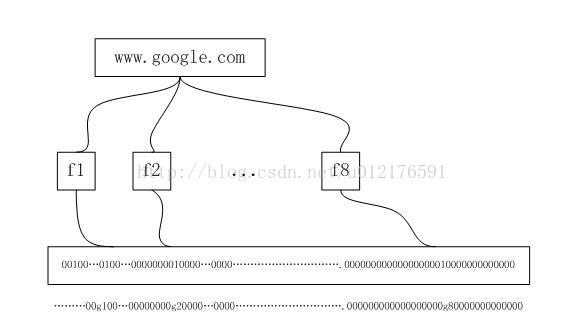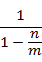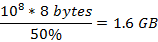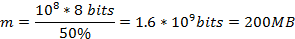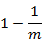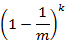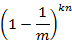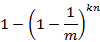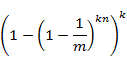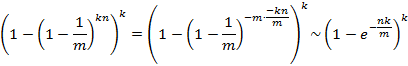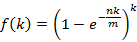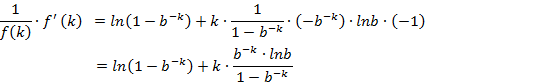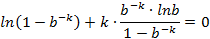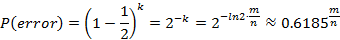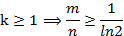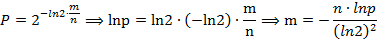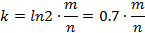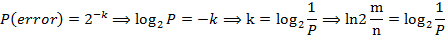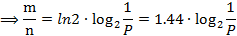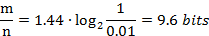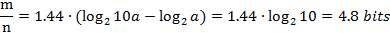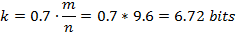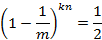package pri.xiaoye.day1029;

import java.io.Serializable;
import java.nio.charset.Charset;
import java.security.MessageDigest;
import java.security.NoSuchAlgorithmException;
import java.util.BitSet;
import java.util.Collection;

/**
* 布隆过滤器的实现类
* 定义：http://en.wikipedia.org/wiki/Bloom_filter
*
* @param <E>
* 		   E指定了要插入过滤器中的元素的类型，eg，String Integer
* @translator xiaoye
*/
public class BloomFilter<E> implements Serializable {

private static final long serialVersionUID = -2326638072608273135L;
private BitSet bitset;
private int bitSetSize;
private double bitsPerElement;
private int expectedNumberOfFilterElements;//能够添加的元素的最大个数
private int k; // 哈希函数的个数

static final Charset charset = Charset.forName("UTF-8");//存储哈希值的字符串的编码方式

static final String hashName = "MD5"; //在大多数情况下，MD5提供了较好的散列精确度。如有必要，可以换成 SHA1算法
static final MessageDigest digestFunction;//MessageDigest类用于为应用程序提供信息摘要算法的功能，如 MD5 或 SHA 算法
static { // 初始化 MessageDigest 的摘要算法对象
MessageDigest tmp;
try {
tmp = java.security.MessageDigest.getInstance(hashName);
} catch (NoSuchAlgorithmException e) {
tmp = null;
}
digestFunction = tmp;
}

/**
* 构造一个空的布隆过滤器. 过滤器的长度为c*n
* @param c
*            表示每个元素占有多少位
* @param n
*            表示过滤器能添加的最大元素数量
* @param k
*            表示需要使用的哈希函数的个数
*/
public BloomFilter(double c, int n, int k) {
this.expectedNumberOfFilterElements = n;
this.k = k;
this.bitsPerElement = c;
this.bitSetSize = (int) Math.ceil(c * n);
this.bitset = new BitSet(bitSetSize);
}

/**
* 构造一个空的布隆过滤器。最优哈希函数的个数将由过滤器的总大小和期望元素个数来确定。
*
* @param bitSetSize
*            指定了过滤器的总大小
* @param expectedNumberOElements
*            指定了过滤器能添加的最大的元素数量
*/
public BloomFilter(int bitSetSize, int expectedNumberOElements) {
this(bitSetSize / (double) expectedNumberOElements,  expectedNumberOElements, (int) Math.round((bitSetSize / (double) expectedNumberOElements)* Math.log(2.0)));
}

/**
* 通过指定误报率来构造一个过滤器。
* 每个元素所占的位数和哈希函数的数量会根据误报率来得出。
*
* @param falsePositiveProbability
*            所期望误报率.
* @param expectedNumberOfElements
*            要添加的元素的数量
*/
public BloomFilter(double falsePositiveProbability, int expectedNumberOfElements) {
this(Math.ceil(-(Math.log(falsePositiveProbability) / Math.log(2)))/ Math.log(2), // c = k/ln(2)
expectedNumberOfElements,
(int) Math.ceil(-(Math.log(falsePositiveProbability) / Math.log(2)))); // k = ln(2)m/n
}

/**
* 根据旧过滤器的数据，重新构造一个新的过滤器
*
* @param bitSetSize
*            指定了过滤器所需位的大小
* @param expectedNumberOfFilterElements
*            指定了过滤器所能添加的元素的最大数量
*            to contain.
* @param actualNumberOfFilterElements
*            指定了原来过滤器的数据的数量
*            <code>filterData</code> BitSet.
* @param filterData
*            原有过滤器中的BitSet对象
*/
public BloomFilter(int bitSetSize, int expectedNumberOfFilterElements,
int actualNumberOfFilterElements, BitSet filterData) {
this(bitSetSize, expectedNumberOfFilterElements);
this.bitset = filterData;
}

/**
* 根据字符串的内容生成摘要
*
* @param val
*            字符串的内容
* @param charset
*            输入数据的编码方式
* @return    输出为一个long类型
*/
public static long createHash(String val, Charset charset) {
return createHash(val.getBytes(charset));
}

/**
* 根据字符串内容生成摘要
*
* @param val
*            指定了输入的字符串。默认的编码为 UTF-8
* @return 输出为一个long类型
*/
public static long createHash(String val) {
return createHash(val, charset);
}

/**
* 根据字节数组生成摘要
*
* @param data
*            输入数据
* @return 输出为long类型的摘要
*/
public static long createHash(byte[] data) {
long h = 0;
byte[] res;

synchronized (digestFunction) {
res = digestFunction.digest(data);
}

for (int i = 0; i < 4; i++) {
h <<= 8;
h |= ((int) res[i]) & 0xFF;
}
return h;
}

/**
* 重写equals方法
*/
@Override
public boolean equals(Object obj) {
if (obj == null) {
return false;
}
if (getClass() != obj.getClass()) {
return false;
}
final BloomFilter<E> other = (BloomFilter<E>) obj;
if (this.expectedNumberOfFilterElements != other.expectedNumberOfFilterElements) {
return false;
}
if (this.k != other.k) {
return false;
}
if (this.bitSetSize != other.bitSetSize) {
return false;
}
if (this.bitset != other.bitset
&& (this.bitset == null || !this.bitset.equals(other.bitset))) {
return false;
}
return true;
}

/**
* 重写了hashCode方法
*
*/
@Override
public int hashCode() {
int hash = 7;
hash = 61 * hash + (this.bitset != null ? this.bitset.hashCode() : 0);
hash = 61 * hash + this.expectedNumberOfFilterElements;
hash = 61 * hash + this.bitSetSize;
hash = 61 * hash + this.k;
return hash;
}

/**
* 根据最大元素数量和过滤器的大小来计算误报率。
* 方法的返回值为误报率。如果插入的元素个数小于最大值，则误报率会比返回值要小。
*
* @return 期望的误报率.
*/
public double expectedFalsePositiveProbability() {
return getFalsePositiveProbability(expectedNumberOfFilterElements);
}

/**
* 通过插入的元素数量和过滤器容器大小来计算实际的误报率。
*
* @param numberOfElements
*            插入的元素的个数.
* @return 误报率.
*/
public double getFalsePositiveProbability(double numberOfElements) {
// (1 - e^(-k * n / m)) ^ k
return Math.pow((1 - Math.exp(-k * (double) numberOfElements
/ (double) bitSetSize)), k);

}

/**
* 通过实际插入的元素数量和过滤器容器大小来计算实际的误报率。
*
* @return 误报率.
*/
public double getFalsePositiveProbability() {
}

/**
* 返回哈希函数的个数 k
*
* @return  k.
*/
public int getK() {
return k;
}

/**
* 清空过滤器元素
*/
public void clear() {
bitset.clear();
}

/**
* 向过滤器中添加元素。
* 添加的元素的toString()方法将会被调用，返回的字符串作为哈希函数的输出。
*
* @param element
*            要添加的元素
*/
long hash;
String valString = element.toString();
for (int x = 0; x < k; x++) {
hash = createHash(valString + Integer.toString(x));
hash = hash % (long) bitSetSize;
bitset.set(Math.abs((int) hash), true);
}
}

/**
* 添加一个元素集合到过滤器中
*
* @param c
*            元素集合.
*/
public void addAll(Collection<? extends E> c) {
for (E element : c)
}

/**
* 用来判断元素是否在过滤器中。如果已存在，返回 true。
*
* @param element
*            要检查的元素.
* @return 如果估计该元素已存在，则返回true
*/
public boolean contains(E element) {
long hash;
String valString = element.toString();
for (int x = 0; x < k; x++) {
hash = createHash(valString + Integer.toString(x));
hash = hash % (long) bitSetSize;
if (!bitset.get(Math.abs((int) hash)))
return false;
}
return true;
}

/**
* 判断一个集合中的元素是否都在过滤器中。
*
* @param c
*            要检查的元素集合
* @return 如果集合所有的元素都在过滤器中，则返回true。
*/
public boolean containsAll(Collection<? extends E> c) {
for (E element : c)
if (!contains(element))
return false;
return true;
}

/**
* 得到某一位的值
*
* @param bit
*            bit的位置.
* @return 如果该位被设置，则返回true。
*/
public boolean getBit(int bit) {
return bitset.get(bit);
}

/**
* 设置过滤器某一位的值
*
* @param bit
*            要设置的位置.
* @param value
*            true表示已经成功设置。false表示改为被清除。
*/
public void setBit(int bit, boolean value) {
bitset.set(bit, value);
}

/**
* 返回存放信息的位数组.
*
* @return 位数组.
*/
public BitSet getBitSet() {
return bitset;
}

/**
* 得到过滤器中位数组个大小。
*
* @return 数组大小.
*/
public int size() {
return this.bitSetSize;
}

/**
* 返回已添加的元素的个数
*
* @return 元素个数.
*/
public int count() {
}

/**
* 得到能添加的元素的最大数量
*
* @return  最大数量.
*/
public int getExpectedNumberOfElements() {
return expectedNumberOfFilterElements;
}

/**
* 得到每个元素占用的位的个数的期望值
*
* @return 每个元素占用的位数
*/
public double getExpectedBitsPerElement() {
return this.bitsPerElement;
}

/**
* 得到每个元素占用位数的实际值
*
* @return 每个元素占用的位数.
*/
public double getBitsPerElement() {
}
}

©️2019 CSDN 皮肤主题: 大白 设计师: CSDN官方博客